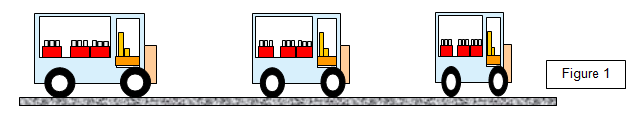# The equations of special relativity

Consider an object of rest mass mo and length Lo, moving with velocity v relative to a stationary frame of reference.

The following equations give the mass m, length L and time t as measured from the frame of reference.The variation of mass with speed shows the ultimate cosmic speed limit. As objects get faster and faster they seem to an outside observer to get heavier and heavier – they are therefore more difficult to accelerate, a fact found in the high- energy particle accelerators.

m = mo/[1-v2/c2]˝
The faster the object moves relative to the observer the greater is its mass

L = Lo[1-v2/c2]˝
The faster the object moves relative to the observer the smaller is its length

t = to/[1-v2/c2]˝
The faster the object moves relative to the observer the slower time passes

The quantity 1/[1-v2/c2]1/2 or [1-v2/c2]-1/2 is often written as γ. The equations can therefore be written as:

m = γ mo
L = Lo
t = γ to

Example problem
An interstellar starship travels through space at high speed. Find the mass of an object with a rest mass of 1 kg, the length of a bar of rest length 1 m and the new value of the second when all these quantities are measured relative to a frame of reference at rest outside the starship.
Consider three cases:
(a) the starship has a velocity of 104 ms-1         γ = 1.000000001 effectively 1
(b) the starship has a velocity of 2x108 ms-1     g = 1.342
(c) the starship has a velocity of 2.7x108 ms-1   γ = 2.294

Using the above equations we have:
(a) m = 1.00 kg, L = 1.00m, t= 100 s
(b) m = 1.34 kg, L = 0.75m, t= 1.34 s
(c) m = 2.29 kg, L = 0.44m, t = 2.29 s.